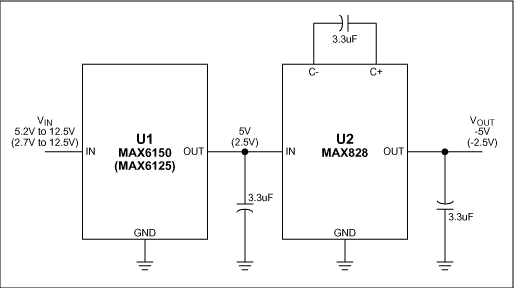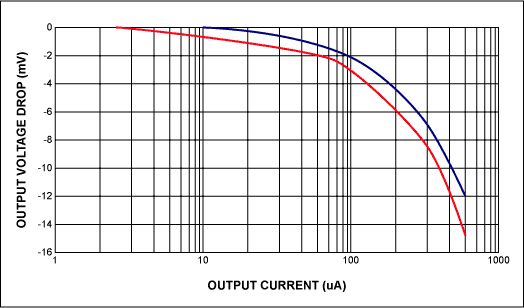# Miniature, Precision Negative Reference Requires No Precision Resistors

### 要約

This application note discusses how to build a negative voltage reference without using external resistors or a negative supply by simply combining a simple charge-pump inverter and a positive output voltage reference.

Switched-capacitor charge-pumps, which occupy less space than do their inductor-based counterparts, are popular for providing inverted voltages in small hand-held equipment. Combining a switched-capacitor charge-pump with a precision reference, for example, yields an inverted reference from a positive power supply (Figure 1). Unlike the more typical combination of a positive 3-terminal reference and an op-amp inverter, this one delivers accurate inversions without the need for precision resistors and a negative supply.Figure 1. A precision negative reference.

The charge-pump inverter (U2) delivers -5V by inverting the output of a 5V precision reference (U1). U1 accepts inputs between 5.2V and 12.5V. Replacing it with a 2.5V reference that accepts 2.7V to 12.5V, as in the MAX6125, produces a -2.5V output. This circuit is very compact. It needs only three surface-mount capacitors, and the ICs occupy tiny SOT23 packages.

Output-voltage accuracy depends partly on the initial accuracy of U1. The MAX6150/MAX6125 devices, for example, offer 1% accuracy. To this you must add the error due to the circuit's output voltage drop, which is less than 2mV for 90µA of load current (Figure 2).Figure 2. The load-regulation error of the circuit's output.

For a -2.5V output, the circuit draws low quiescent currents that range from 86µA for a 2.7V input to 105µA for a 12.5V input. For -5V outputs, the circuit draws 127µA for 5.2V inputs and 140µA for 12.5V inputs (Figure 3).Figure 3. The circuit's supply current as a function of load current.

A similar version of this article appeared in the June 10, 1999 issue of EDN magazine.